# fir1

Window-based FIR filter design

## Syntax

b = fir1(n,Wn)
b = fir1(n,Wn,ftype)
b = fir1(___,window)
b = fir1(___,scaleopt)

## Description

example

b = fir1(n,Wn) uses a Hamming window to design an nth-order lowpass, bandpass, or multiband FIR filter with linear phase. The filter type depends on the number of elements of Wn.

example

b = fir1(n,Wn,ftype) designs a lowpass, highpass, bandpass, bandstop, or multiband filter, depending on the value of ftype and the number of elements of Wn.

example

b = fir1(___,window) designs the filter using the vector specified in window and any of the arguments from previous syntaxes.
b = fir1(___,scaleopt) additionally specifies whether or not the magnitude response of the filter is normalized.Note:   Use fir2 for windowed filters with arbitrary frequency response.

## Examples

collapse all

Design a 48th-order FIR bandpass filter with passband $0.35\pi \le \omega \le 0.65\pi$ rad/sample. Visualize its magnitude and phase responses.

b = fir1(48,[0.35 0.65]); freqz(b,1,512)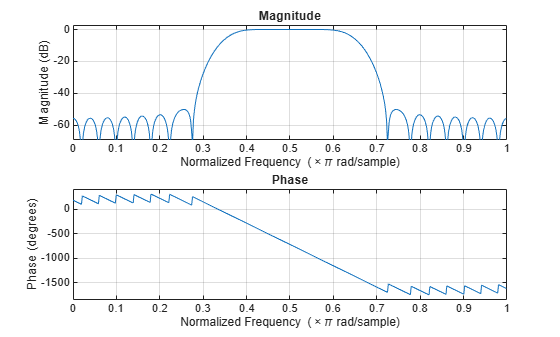Load chirp.mat. The file contains a signal, y, that has most of its power above Fs/4, or half the Nyquist frequency. The sample rate is 8192 Hz.

Design a 34th-order FIR highpass filter to attenuate the components of the signal below Fs/4. Use a cutoff frequency of 0.48 and a Chebyshev window with 30 dB of ripple.

load chirp t = (0:length(y)-1)/Fs; bhi = fir1(34,0.48,'high',chebwin(35,30)); freqz(bhi,1)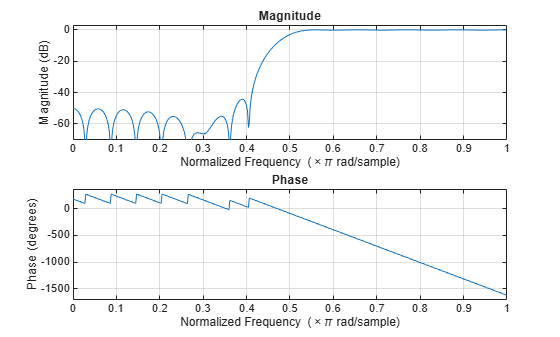Filter the signal. Display the original and highpass-filtered signals. Use the same y-axis scale for both plots.

outhi = filter(bhi,1,y); subplot(2,1,1) plot(t,y) title('Original Signal') ys = ylim; subplot(2,1,2) plot(t,outhi) title('Highpass Filtered Signal') xlabel('Time (s)') ylim(ys)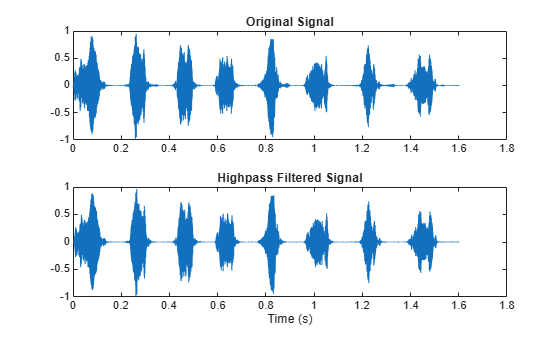Design a lowpass filter with the same specifications. Filter the signal and compare the result to the original. Use the same y-axis scale for both plots.

blo = fir1(34,0.48,chebwin(35,30)); outlo = filter(blo,1,y); subplot(2,1,1) plot(t,y) title('Original Signal') ys = ylim; subplot(2,1,2) plot(t,outlo) title('Lowpass Filtered Signal') xlabel('Time (s)') ylim(ys)Design a 46th-order FIR filter that attenuates normalized frequencies below $0.4\pi$ rad/sample and between $0.6\pi$ and $0.9\pi$ rad/sample. Call it bM. Compute its frequency response.

ord = 46; low = 0.4; bnd = [0.6 0.9]; bM = fir1(ord,[low bnd]); [hbM,f] = freqz(bM,1);

Redesign bM so that it passes the bands it was attenuating and stops the other frequencies. Call the new filter bW. Display the frequency responses of the filters.

bW = fir1(ord,[low bnd],"DC-1"); [hbW,~] = freqz(bW,1); plot(f/pi,mag2db(abs(hbM)),f/pi,mag2db(abs(hbW))) legend("bM","bW",Location="best") ylim([-75 5]) grid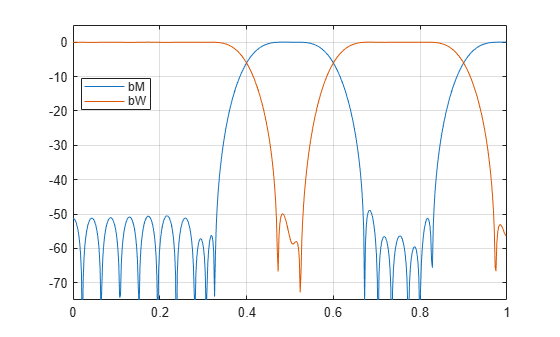Redesign bM using a Hann window. (The "DC-0" is optional.) Compare the magnitude responses of the Hamming and Hann designs.

hM = fir1(ord,[low bnd],'DC-0',hann(ord+1)); hhM = freqz(hM,1); plot(f/pi,mag2db(abs(hbM)),f/pi,mag2db(abs(hhM))) legend("Hamming","Hann",Location="northwest") ylim([-75 5]) grid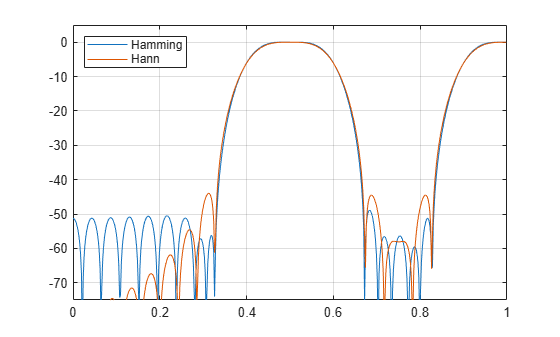Redesign bW using a Tukey window. Compare the magnitude responses of the Hamming and Tukey designs.

tW = fir1(ord,[low bnd],'DC-1',tukeywin(ord+1)); htW = freqz(tW,1); plot(f/pi,mag2db(abs(hbW)),f/pi,mag2db(abs(htW))) legend("Hamming","Tukey",Location="best") ylim([-75 5]) grid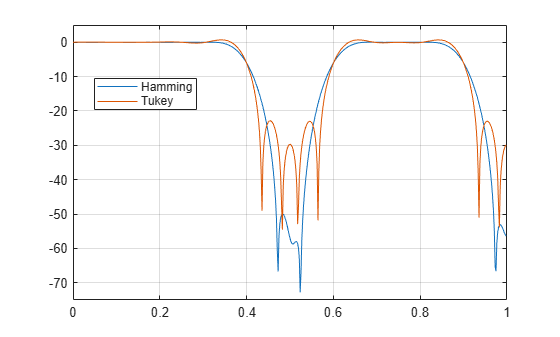## Input Arguments

collapse all

Filter order, specified as an integer scalar.

For highpass and bandstop configurations, fir1 always uses an even filter order. The order must be even because odd-order symmetric FIR filters must have zero gain at the Nyquist frequency. If you specify an odd n for a highpass or bandstop filter, then fir1 increments n by 1.

Data Types: double

Frequency constraints, specified as a scalar, a two-element vector, or a multi-element vector. All elements of Wn must be strictly greater than 0 and strictly smaller than 1, where 1 corresponds to the Nyquist frequency: 0 < Wn < 1. The Nyquist frequency is half the sample rate or π rad/sample.

• If Wn is a scalar, then fir1 designs a lowpass or highpass filter with cutoff frequency Wn. The cutoff frequency is the frequency at which the normalized gain of the filter is –6 dB.

• If Wn is the two-element vector [w1 w2], where w1 < w2, then fir1 designs a bandpass or bandstop filter with lower cutoff frequency w1 and higher cutoff frequency w2.

• If Wn is the multi-element vector [w1 w2 ... wn], where w1 < w2 < … < wn, then fir1 returns an nth-order multiband filter with bands 0 < ω < w1, w1 < ω < w2,  …, wn < ω < 1.

Data Types: double

Filter type, specified as one of the following:

• 'low' specifies a lowpass filter with cutoff frequency Wn. 'low' is the default for scalar Wn.

• 'high' specifies a highpass filter with cutoff frequency Wn.

• 'bandpass' specifies a bandpass filter if Wn is a two-element vector. 'bandpass' is the default when Wn has two elements.

• 'stop' specifies a bandstop filter if Wn is a two-element vector.

• 'DC-0' specifies that the first band of a multiband filter is a stopband. 'DC-0' is the default when Wn has more than two elements.

• 'DC-1' specifies that the first band of a multiband filter is a passband.

Window, specified as a vector. The window vector must have n + 1 elements. If you do not specify window, then fir1 uses a Hamming window. For a list of available windows, see Windows.

fir1 does not automatically increase the length of window if you attempt to design a highpass or bandstop filter of odd order.

Example: kaiser(n+1,0.5) specifies a Kaiser window with shape parameter 0.5 to use with a filter of order n.

Example: hamming(n+1) is equivalent to leaving the window unspecified.

Data Types: double

Normalization option, specified as either 'scale' or 'noscale'.

• 'scale' normalizes the coefficients so that the magnitude response of the filter at the center of the passband is 1 (0 dB).

• 'noscale' does not normalize the coefficients.

## Output Arguments

collapse all

Filter coefficients, returned as a row vector of length n + 1. The coefficients are sorted in descending powers of the Z-transform variable z:

B(z) = b(1) + b(2)z + … + b(n+1)z–n.

## Algorithms

fir1 uses a least-squares approximation to compute the filter coefficients and then smooths the impulse response with window.

 Digital Signal Processing Committee of the IEEE Acoustics, Speech, and Signal Processing Society, eds. Programs for Digital Signal Processing. New York: IEEE Press, 1979, Algorithm 5.2.